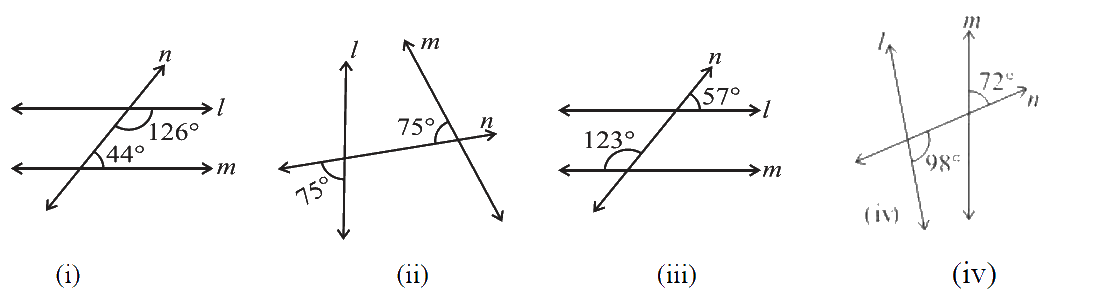# Ex.5.2 Q6 Lines and Angles - NCERT Maths Class 7

## Question

In the given figures below, decide whether $$l$$  is parallel to $$m.$$Video Solution
Lines & Angles
Ex 5.2 | Question 6

## Text Solution

(i) Reasoning:

Let’s visually model this problem. There is one operation that can be done check whether interior angles are supplementary or not. According to this model, the result sum of $$126^\circ + 44^\circ$$ is $$170^\circ.$$ Now, it’s a matter of finding $$l$$ is parallel to $$m$$ or not

Steps:

(i) $$126^\circ + 44^\circ = 170^\circ$$

As the sum of interior angles on the same side of transversal $$n$$ is not $$180^\circ.$$

Therefore, $$l$$ is not parallel to $$m.$$

(ii) Reasoning:

Let’s visually model this problem. There are two operations that can be done in a sequence. First find the value of $$x$$ and then check it is equal to its corresponding angle or not. According to this model, the resultant value of $$x$$ is not equal to its corresponding angle. Now, it’s a matter of finding $$l$$ is parallel to $$m$$ or not

(ii) Steps:

\begin{align}\angle x + 75^\circ &= 180^\circ \text{(Linear pair)}\\\angle x &= 180^\circ - 75^\circ\\\angle x &= 105^\circ\end{align}

For $$l$$ and $$m$$ to be parallel measure of their corresponding angles should be equal but here the measure of $$\angle x$$ is $$105^\circ$$ and its corresponding angle is $$75^\circ.$$

Therefore, the lines $$l$$ and $$m$$ are not parallel.

(iii) Reasoning:

Let’s visually model this problem. There are two operations that can be done in a sequence. First find the value of $$x$$ and then check it is equal to its corresponding angle or not. According to this model, the resultant value of $$x$$ is not equal to its corresponding angle. Now, it’s a matter of finding $$l$$ is parallel to $$m$$ or not

(iii) Steps:

\begin{align}\angle y&=57^\circ \begin{bmatrix}\text{Vertically opposite}\\\text {angles}\end{bmatrix}\\\angle x + 123^\circ &= 180^\circ\text{ (Linear pair)}\\\angle x& = 180^\circ - 123^\circ\\\angle x &= 57^\circ\end{align}

Here, the measure of corresponding angles are equal i.e $$57^\circ.$$

Therefore, lines l and m are parallel to each other.

(iv) Reasoning:

Let’s visually model this problem. There are two operations that can be done in a sequence. First find the value of $$x$$ by using linear pairand then check it is equal to its corresponding angle or not. According to this model, the resultant value of $$x$$ is not equal to its corresponding angle. Now, it’s a matter of finding $$l$$ is parallel to $$m$$ or not

(iv) Steps:

\begin{align}\angle x + 98^\circ &= 180^\circ \text{(Linear pair)}\\\angle x &= 180^\circ - 98^\circ\\\angle x &= 82^\circ\end{align}

For $$l$$ and $$m$$ to be parallel measure of their corresponding angles should be equal but here the measure of corresponding angles are $$82^\circ$$ and $$72^\circ$$ whichn are not equal.

Therefore, $$l$$ and $$m$$ are not parallel to each other.

Learn from the best math teachers and top your exams

• Live one on one classroom and doubt clearing
• Practice worksheets in and after class for conceptual clarity
• Personalized curriculum to keep up with school